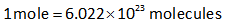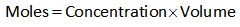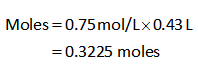Question
1 views

How many moles of NaCl are in 0.43 L of a 0.75 M NaCl solution?

check_circle

Introduction

Mole concept is applied to find out the quantity of any compound in moles. The moles can be analyzed with the given solution's concentration and volume. A mole is defined by Avogadro number i.e.Given

The concentration of NaCl solution is 0.75 M.

The volume of NaCl solution is 0.43 L.

Calculation

The formula for the calculation of the number of moles is shown below.Substitute the known values in the formula.Thus the number of moles of NaCl is 0.3225 moles.

...

### Want to see the full answer?

See Solution

#### Want to see this answer and more?

Solutions are written by subject experts who are available 24/7. Questions are typically answered within 1 hour.*

See Solution
*Response times may vary by subject and question.
Tagged in